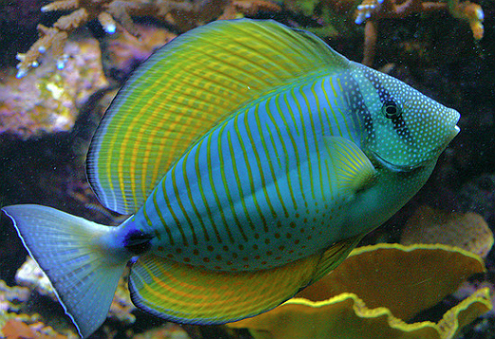# Silhouette of a Fish

Calculus Level 3Find the minimum possible value of $|a|$ such that the function below is continuous along the interval $[b,\infty)$ and there is no value of $x such that $y$ is a real number.

$16y^2=a+15 x-9 x^2+x^3$

Note: $b$ is a constant of real integral value.

###### Image Credit: Flickr Larry
×

Problem Loading...

Note Loading...

Set Loading...